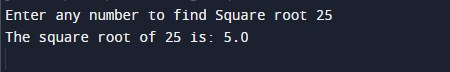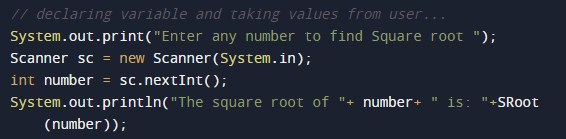# Java Program to find square root of a number without sqrt method

In this tutorial you will learn about the Java Program to find square root of a number without sqrt method and its application with practical example.

In this tutorial, we will learn to create a Java Program to find square root of a number without sqrt method using Java programming.

## Prerequisites

Before starting with this tutorial we assume that you are best aware of the following Java programming topics:

• Java Operators.
• Basic Input and Output function in Java.
• Class and Object in Java.
• Basic Java programming.
• If-else statements in Java.
• For loop in Java.
• User Define functions

## What is square root?

Square root  of number that we multiply the number by itself to get the original number.Rather we can say that  “square root of a number” is the value of power 1/2 of that number.

It is  symbolized by‘√ ‘.
Square root of 625 = 25.

We are going to use following Formula to find the square root of a number.

sqrt=(sqrt_num+(number/sqrt_num))/2;

where sqrt_num is agiven number by user…

## Java Program to find square root of a number without sqrt method

In this program we would find the Square root  of a given number .First of all we take a vaue form user and find the Square root of a number.Let have a look at the code.

## Output

Square root of a number.In the above program, we have first declared and initialized a set variables required in the program.

• number= it will hold entered number.
• temp = it will hold temporary number.
• sqrt=  it will hold the result.

After declaring variables we take a value from user and find Square root of a number..after getting value from user we pass this value to a method named Sroot(number) where we calculate Square root of  a number.this function calculates square root and return the square root a provided number and we will print the result.In this tutorial we have learn about the Java Program to find square root of a number without sqrt method and its application with practical example. I hope you will like this tutorial.